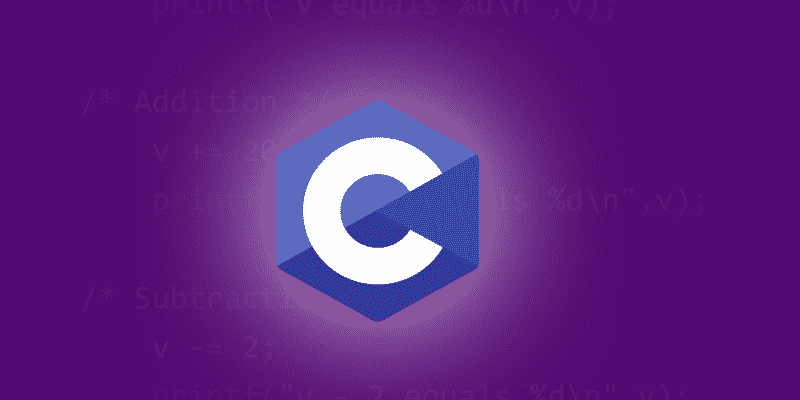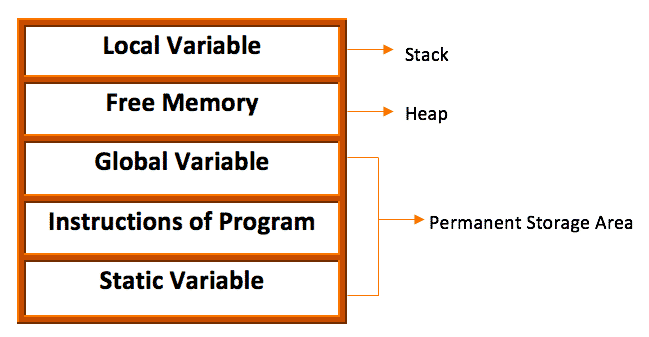ProgrammingTech Terms

# Dynamic Memory Allocation in C Programming Language

Dynamic memory allocation is one of the important and core concepts in “C” and also, one of the nipping topics for the point of interviews. Malloc, Calloc, Free, and Realloc comes under the “STDLIB.H” header files in “C” and are basically the functions used in the implementation of Dynamic memory allocation.

In this article, we will focus on these functions and will try to understand how to use these functions efficiently. So, let us dive into the topic.

### What is Dynamic Memory Allocation?

The process of allocating memory at runtime is known as dynamic memory allocation. What does this mean “at runtime”? Generally, when we declare a primitive variable suppose “int a;”  then memory is allocated at the compile time and we call that Static memory” allocation. By static, we mean that the memory that is been allocated is actually fixed in size.

If we talk about the array, then the declared size is also fixed so this even comes under the static memory allocation. Moreover, user-defined datatype for example structure size is also fixed when we hard code our program.

These kinds of the declaration have fixed size initially something which is not preferable and we don’t want hard-coded program each and every time. We need something that should be dynamic and according to the requirements, the allocation of memory should be done.

There can be various scenarios when we need extra memory or the memory of the program is not specified beforehand so, it is dynamically allocated according to the need of the program. For example, in the case of an array, the size can be increased or decreased based on the elements that we are storing and deleting.

### How Do We Achieve The Dynamic memory Allocation?

In case of dynamic allocation, library routines known as memory management functions are used for allocating and freeing memory during execution of a program. These functions are defined in “stdlib.h” header file. This provides the necessary functions to allocate and deallocate the memory that we will discuss later in this post.

In order to understand the dynamic memory allocation better, let us first concentrate on how memory is distributed among various parameters in a “C” program. Observe the below table.Generally, Global variables, Static variables, and Programs Instructions are stored in the permanent storage area. Whereas, local variables are stored in a memory area called Stack.

The memory between the local and global variable is called as Heap area. It is basically a data structure used for dynamic memory allocation during execution of our program. The size of the heap keeps on changing as per the memory requirements.

### Functions Used while implementing Dynamic memory Allocation

##### 1. malloc() Function

This function is used to allocated memory to arrays or structures” but for the single block of requested memory. Basically, this method is used to allocate the memory for the user-defined data types in C language. This function reserves a block of memory of specified size and returns a pointer of type “void” which can be casted into a pointer of any form. What do we mean by this line? So,

In dynamic allocation, pointers play a key role.  The function takes an argument i.e the size that how many bytes we want to allocate and then return type is a “void-pointer” which is a generic type” and according to our convenience we can type cast it in whatever we want i.e.  it could be type-casted in an integer, char, double, float, struct, linked list, graph node or anything.

Ones the memory is allocated successfully, it returns the base address of the memory that our returned pointers hold and on failure, it returns the “null pointer”.

``SYNTAX:- void* malloc(byte-size);``

When malloc function is executed, then depending upon the compiler and 16 or 32 bit OS, it accordingly allocates the memory for the datatypes.

Example Program:

``````#include <stdio.h>
#include <stdlib.h>
#include <conio.h>
void main()
{
int number, i, *pointer, sum = 0;
printf("Enter number of elements: ");
scanf("%d", &number);

// memory allocation using the malloc function
pointer = (int*) malloc(number * sizeof(int));
// here (int*) is actually typecasted from void pointer
// to integer pointer since our function accept the integer type.

if(pointer == NULL)
{
printf("Memory not allocated.");
exit(0);
}
printf("Enter elements of array: ");
for(i = 0; i < number; ++i)
{
scanf("%d", pointer + i);
sum += *(pointer + i);
}
printf("Sum = %d", sum);
free(pointer);
getch();
}``````
##### 2. calloac() Function

This method is also used to allocate memory to arrays and structures. Like malloc, if it fails to allocate enough space as specified, it returns a null pointer. The biggest difference between the malloc() and calloac() is that it handles the multiple requests for the memory allocation.

`SYNTAX:-  (void*) calloc(number_of_items, element_size);`

The function takes two arguments, one is the size of the array or say the datatype and the other is the size of each element and the return type is again a void pointer that is a generic type. If sufficient memory is available then calloac function allocates a memory block of size “number of items * size of elements” and returns the base address stored in the void pointer.

Example Program:

``````#include <stdio.h>
#include <stdlib.h>
#include <conio.h>
void main()
{
int number, i, *ptr, sum = 0;
printf("Enter number of elements: ");
scanf("%d", &number);
// implementing the calloc function
ptr = (int*) calloc(number, sizeof(int));
if(ptr == NULL)
{
printf("Error! memory not allocated.");
exit(0);
}

printf("Enter elements of array: ");
for(i = 0; i < number; ++i)
{
scanf("%d", ptr + i);
sum += *(ptr + i);
}

printf("Sum = %d", sumber);
free(ptr);
getch();
}``````
```Note:- If the first argument of the calloc() function is null then
it is equivalent to malloc() function.```
##### 3. realloc() function

The basic motto of this method is to increase or decrease the size of an array or say reallocate the memory that has been allocated by the malloc() and calloc() functions if the previously allocated memory is insufficient or more than required.

`SYNTAX:- ptr = realloc(ptr, newsize);`

Here, in the arguments,  first, we have the pointer to the starting address of the existing block and second argument is the size of the new block which can be greater or smaller so that you can specify the new size.

Example Program:

``````#include <stdio.h>
#include <stdlib.h>
#include <conio.h>
void main()
{
int *ptr, i , n1, n2;
printf("Enter size of array: ");
scanf("%d", &n1);

ptr = (int*) malloc(n1 * sizeof(int));

printf("Address of previously allocated memory: ");
for(i = 0; i < n1; ++i)
printf("%u\t",ptr + i);

printf("\nEnter new size of array: ");
scanf("%d", &n2);
// reallocating the memory of size n2
ptr = realloc(ptr, n2 * sizeof(int));
for(i = 0; i < n2; ++i)
printf("%u\t", ptr + i);
getch();
}``````
##### 4. free function

Dynamically allocated memory created using the calloc() or malloc() methods don’t get free on its own. We need to explicitly use free() method to release the space. So, when we need to delete or release the memory then, the “free function” is called.

`SYNTAX:- free(ptr);`Research Intern, INSA Rouen | Technical Writer, Self-learner and Tech exuberant person.

## 1 Comment

1.Ajay00100 says:

thnx…
Sir, tell me how to get Internship in top companies, as a full stack Developer.
every daily posts are very useful for me…I m waiting everyday.

This site uses Akismet to reduce spam. Learn how your comment data is processed.